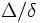# Pinching constant

The pinching constant for a Riemannian metric on a (compact) differential manifold is defined as the ratio$\Delta/\delta$ where$\Delta$ and$\delta$ are, respectively, the maximum and minimum values taken by sectional curvatures for tangent planes on the manifold.